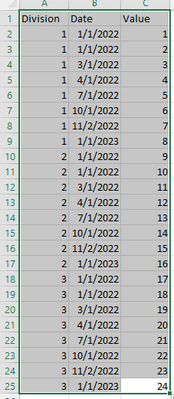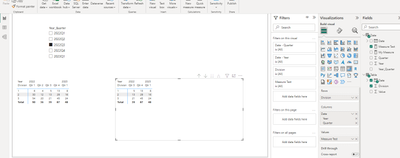cancel
Showing results for
Did you mean:Anonymous
Not applicable

## Use slicer to filter column

I want to use my quarter year column as a slicer, when I select a single quarter year, the resulting outcome in the matrix table should give me the output as 'Selected quarter from the slicer and next two quarters' as shown in the below image. Can someone suggest any method or know the proper DAX for the following outcome?1 ACCEPTED SOLUTIONCommunity Support

Hi , @Anonymous

According to your description, you want to "Use slicer to filter column".

Here are the steps you can refer to:
(1)This is my test data:(2)We can click "New Table" to  create a date  table as a slicer:

``````Date = ADDCOLUMNS(
CALENDAR(FIRSTDATE('Table'[Date]),LASTDATE('Table'[Date])),
"Year", YEAR ( [Date] ),
"Quarter", ROUNDUP(MONTH([Date])/3,0),
"Year_Quarter", year([date]) & "Q" & ROUNDUP(MONTH([Date])/3,0)
)``````

And we do not create any relationship between two tables.

And in the raw visual i use a measure to show the value:

My Measure = SUM('Table'[Value])

(3)Then we can create a measure :

``````Measure Test = var _min_quarter_date = MIN('Date'[Date])
var _end_quarter_date = EOMONTH(_min_quarter_date ,8)
var _cur_date = MIN('Table'[Date])
return
IF(_cur_date>=_min_quarter_date && _cur_date <= _end_quarter_date , [My Measure] , BLANK())``````

(4)Then we can put the fields we need on the visual and we can meet your need:Thank you for your time and sharing, and thank you for your support and understanding of PowerBI!

Best Regards,

Aniya Zhang

If this post helps, then please consider Accept it as the solution to help the other members find it more quickly

4 REPLIES 4Community Support

Hi , @Anonymous

According to your description, you want to "Use slicer to filter column".

Here are the steps you can refer to:
(1)This is my test data:(2)We can click "New Table" to  create a date  table as a slicer:

``````Date = ADDCOLUMNS(
CALENDAR(FIRSTDATE('Table'[Date]),LASTDATE('Table'[Date])),
"Year", YEAR ( [Date] ),
"Quarter", ROUNDUP(MONTH([Date])/3,0),
"Year_Quarter", year([date]) & "Q" & ROUNDUP(MONTH([Date])/3,0)
)``````

And we do not create any relationship between two tables.

And in the raw visual i use a measure to show the value:

My Measure = SUM('Table'[Value])

(3)Then we can create a measure :

``````Measure Test = var _min_quarter_date = MIN('Date'[Date])
var _end_quarter_date = EOMONTH(_min_quarter_date ,8)
var _cur_date = MIN('Table'[Date])
return
IF(_cur_date>=_min_quarter_date && _cur_date <= _end_quarter_date , [My Measure] , BLANK())``````

(4)Then we can put the fields we need on the visual and we can meet your need:Thank you for your time and sharing, and thank you for your support and understanding of PowerBI!

Best Regards,

Aniya Zhang

If this post helps, then please consider Accept it as the solution to help the other members find it more quicklyAnonymous
Not applicable

Hey Aniya Zhang,
If we compare our dataset ..you have Value in Numerical, whereas in my data the Values are in String Format. i can't use the 'My Measure' which has the SUM function.. any walkaround for the same ??Community Support

Hi, @Anonymous

If you are using the "String" type of data, please use the "Max()" function:

MAX function (DAX) - DAX | Microsoft LearnSolution Sage

@Anonymous Can you share sample data and sample output in table format? Or a sample pbix after removing sensitive data.